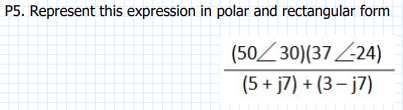Home / Expert Answers / Electrical Engineering / p5-represent-this-expression-in-polar-and-rectangular-form-5-j7-3j7-5030-3724-pa634

# (Solved): P5. Represent this expression in polar and rectangular form (5+j7)+(3j7)(5030)(3724) ...P5. Represent this expression in polar and rectangular form

We have an Answer from Expert

To represent the given expression in polar and rectangular form, let's break it down step by step:-

Given expression:

Step 1: Convert the complex numbers to rectangular form:-
We can use Euler's formula to convert the numbers in polar form to rectangular form:
For   :
For

Step 2: Convert the sum in the denominator to rectangular form:-
We have an Answer from Expert